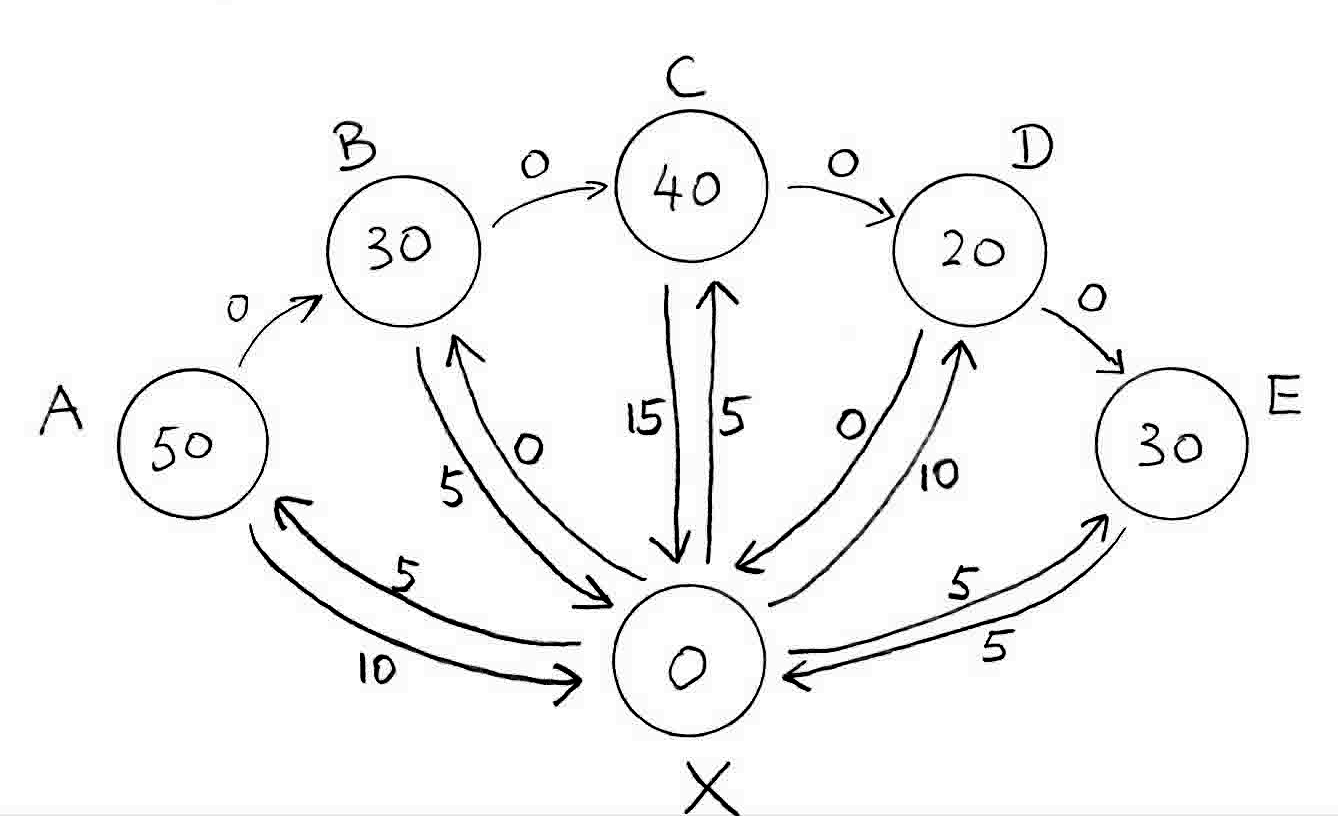## Sunday, March 20, 2016

### Better Time Management for Programmers

Here's a common scheduling problem we, the programmers, will likely face in our career at one point or another.

You have a project or program that will take a long time to finish and you only have a fixed maximum time slot to work on it every day.  The project is structured and divided into a series of smaller tasks or milestones such that:
1. The tasks are ordered and performed sequentially.  This means a following task cannot start until the previous task is finished.

2. The duration of each task differs.  Some tasks take longer than others but each task lasts shorter than the daily time slot allowed.  Some tasks may be so short that it's possible to finish several within the same time slot.

3. Tasks have overhead time penalty.  The first and last task completed in a time slot incur set-up and tear-down time penalty, respectively.  The penalty amount depends on the task.
To illustrate the problem with an example, let's say your project has 5 sequential tasks or milestones - A, B, C, D and E.  You can only work on the project at most 2 hours (120 minutes) a day.  The time it takes to finish each task along with set-up and tear-down penalty time are:

Task Execution Time Set-up Time Tear-down Time
A 50 10 5
B 30 5 0
C 40 15 5
D 20 0 10
E 30 5 5

All time units are in minutes.  Set-up and tear-down time are the time penalty for context switching tasks in and out of the time slot.  In real-life, these could be ceremonious activities such as booting up the machines, push or pulling source code from version control, reading or documenting code, loading or persisting large data set to or from memory, powwows, soaking up chakra, etc.

There are many ways of scheduling the tasks.  Some are valid and some are not.  For example, if you choose task A, B and C on the first day, the set-up time is 10 (A's set-up time).  Tear-down time is 5 (C's tear-down time).  Task A, B and C take 50, 30 and 40 minutes to execute, respectively.  The total time to complete A, B and C is 10 + 50 + 30 + 40 + 5 = 135 minutes.  Since your maximum daily time slot is 120 minutes, this combination exceeds the time slot so it's not a valid choice.

As an example of a valid scheduling, you can assign A and B to the first day, C and D to the second, and E to the third.  The total time to complete the project is:

(A + B) + (C + D) + E = (10 + 50 + 30 + 0) + (15 + 40 + 20 + 10) + (5 + 30 + 5) = 215 minutes

As much as we programmers like to program, we like downtime, too.  The quicker we finish the project the better.  So how do we find the best scheduling assignment?  There is a simple and clever solution to this thanks to Prin et al  from the seemingly unrelated problem domain of vehicle routing.  I'll explain the intuition behind the idea.  For those who are looking for an efficient labeling algorithm implementing the idea, please refer to Prin et al .

First, we'll represent the scheduling problem with a graph:Graphical representation of tasks

In the above graph, there are two types of vertices - task vertex and breakpoint vertex.  Breakpoint vertex X represents the starting and ending point of a time slot.  Task vertex A, B, C, D and E represent the 5 tasks in our example problem.  A directed edge between X and task vertex represents the set-up time of the task while a directed edge between task vertex and X represents the tear-down time of the task.  The edges between tasks represent the time required to transition from one task to another which is 0 in our case.  Lastly, each vertex is weighted with the execution time of the task.  The execution time of vertex X is 0.

In reading of this graph, the schedule of assigning A and B to the first day, C and D to the second, and E to the third, is represented by path XABXCDXEX.  The total project completion time is the sum of edge and vertex weights of the path.

Next, we'll construct a simple auxiliary graph as such: